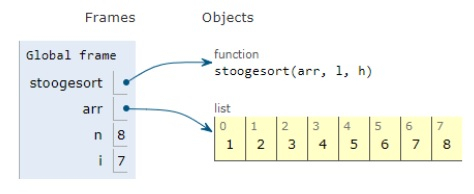# Python Program for Stooge Sort

Problem statement − We are given an array, we need to sort it using stooge sort.

## Algorithm

1. Check if value at index 0 is greater than value at last index,then swap them.
2. sort the initial 2/3rd of the array.
3. sort the last 2/3rd of the array.
4. sort the initial 2/3rd again to confirm.

Now let’s observe the solution in the implementation below −

## Example

Live Demo

def stoogesort(arr, l, h):
if l >= h:
return
# swap
if arr[l]>arr[h]:
t = arr[l]
arr[l] = arr[h]
arr[h] = t
# more than 2 elements
if h-l+1 > 2:
t = (int)((h-l+1)/3)
# sort first 2/3 elements
stoogesort(arr, l, (h-t))
# sort last 2/3 elements
stoogesort(arr, l+t, (h))
# sort first 2/3 elements again
stoogesort(arr, l, (h-t))
# main
arr = [1,4,2,3,6,5,8,7]
n = len(arr)
stoogesort(arr, 0, n-1)
print ("Sorted sequence is:")
for i in range(0, n):
print(arr[i], end = " ")

## Output

Sorted sequence is:
1 2 3 4 5 6 7 8All the variables are declared in the local scope and their references are seen in the figure above.

## Conclusion −

In this article, we have learned about how we can make a Python Program for Stooge Sort